Varsity Tutors always has a different Differential Equations Question of the Day ready at your disposal! If you’re just looking to get a quick review into your busy day, our Differential Equations Question of the Day is the perfect option. Answer enough of our Differential Equations Question of the Day problems and you’ll be ready to ace the next test. Check out what today’s Differential Equations Question of the Day is below.

### Question of the Day: Differential Equations

Given the matrix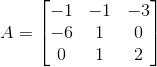, calculate the matrix exponential,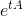. You may leave your answer diagonalized: i.e. it may contain matrices multiplied together and inverted.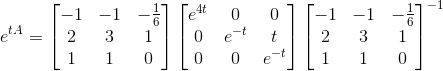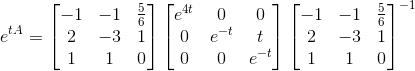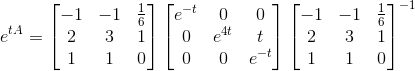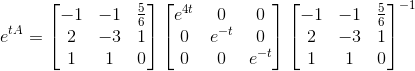### All Differential Equations Resources